﻿ 基于时频联合的非合作水声信号盲检测
 舰船科学技术2023, Vol. 45Issue (1): 157-162    DOI: 10.3404/j.issn.1672-7649.2023.01.028PDF

Blind detection of non-cooperative underwater acoustic signals based on time-frequency domain association
DENG Hong-chao, ZHANG Gang-qiang, LIU Ben-qi
Shanghai Marine Electronic Equipment Research Institute, Shanghai 201108, China
Abstract: Traditional blind detectors confront difficulty in detecting non-cooperative band-limited pulse signals with low SNR. A new method is proposed in this paper. After STFT, extract part of the received data above limits in time-frequency domain and use it as replica to match with the following received data. Outputs of match filter over threshold are used to implement blind detection of the repeated non-cooperative pulse signal hided in noise. It′s performance is better than traditional methods 4 dB approximately and tested in trial data.
Key words: non-cooperative     blind detection     time-frequency analysis     underwater acoustic signal reconnaissance
0 引　言

1 基于时频联合的盲检测方法 1.1 算法流程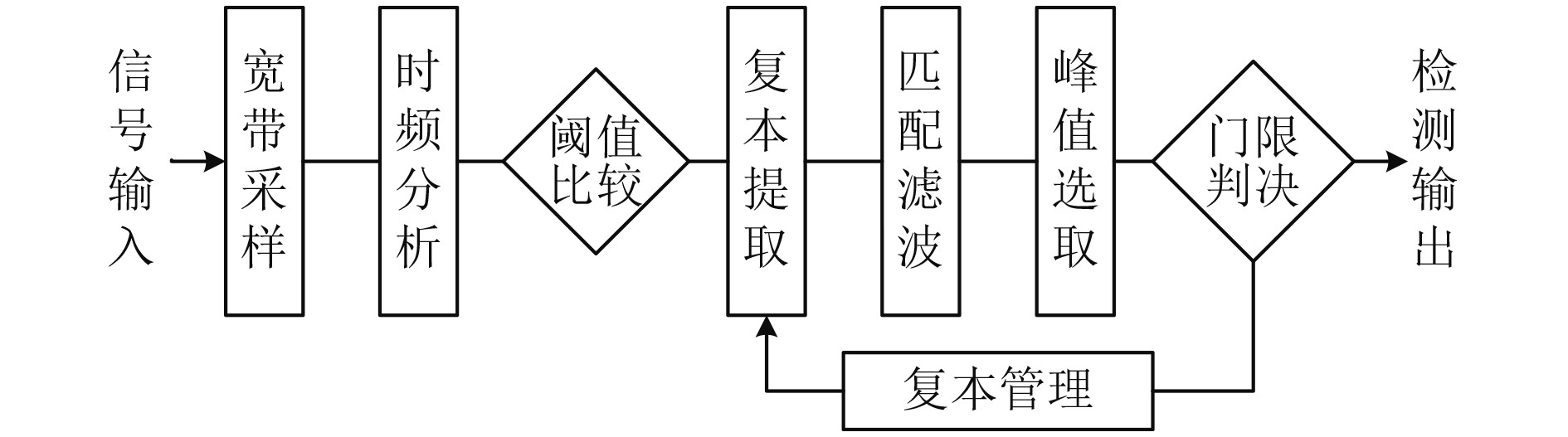图 1 算法流程框图 Fig. 1 Structure diagram of the detection algorithm
1.2 信号模型

1）LFM信号的解析表示为：

 $x\left( t \right) = A{e^{j\left( {2\text{π} {f_0}t +\text{π} k{t^2}} \right)}} ，\begin{array}{*{20}{c}} {}&{t \in \left[ {\begin{array}{*{20}{c}} { - T/2,}&{T/2} \end{array}} \right]}。\end{array}$ (1)

2）HFM信号的解析表示为：

 $x\left( t \right) = A{e^{ - j\left( {2\text{π} \frac{{f_0^2}}{m}} \right)\ln \left( {1 - \frac{m}{{{f_0}}}t} \right)}}，\begin{array}{*{20}{c}} {}&{t \in \left[ {\begin{array}{*{20}{c}} { - T/2,}&{T/2} \end{array}} \right]} 。\end{array}$ (2)

3）直接序列扩频信号可表示为：

 $x\left( t \right) = Ad(t)p(t){e^{j\left( {2\text{π}{f_0}t + {\varphi _0}} \right)}} 。$ (3)

4）混沌序列调频信号的数学表示为：

 $x\left( t \right) = A{e^{j2\text{π} \left[ {{f_0}t + \Delta f\int_0^t {\varsigma \left( \tau \right){\rm{d}}\tau } } \right]}}。$ (4)

1.3 复本提取

1）滑动窗能量检测

 $c(t) = \frac{1}{T}\int_{t - T}^t {{x^2}(u){\rm{d}}u}，$ (5)

 $G = {\left\{ {\frac{T}{{2\int_0^T {\left( {1 - \frac{\tau }{T}} \right)\rho _n^2\left( \tau \right){\rm{d}} \tau } }}} \right\}^{1/2}}。$ (6)

 $G = \sqrt {2TW}，$ (7)

 $c(t) = {{\int_t^{t + T} {{x^2}(u){\rm{d}}u} } \mathord{\left/ {\vphantom {{\int_t^{t + T} {{x^2}(u){\rm{d}}u} } {\int_{t - T}^t {{x^2}(u){\rm{d}}u} }}} \right. } {\int_{t - T}^t {{x^2}(u){\rm{d}}u} }}，$ (8)
 $c{(t)_{\max }} \approx SNR + 1。$ (9)

2）延迟自相关检测

 $c(t) = \frac{1}{T}\int_{t - T}^t {x(u)x(u - {\tau _0}){\rm{d}}u}。$ (10)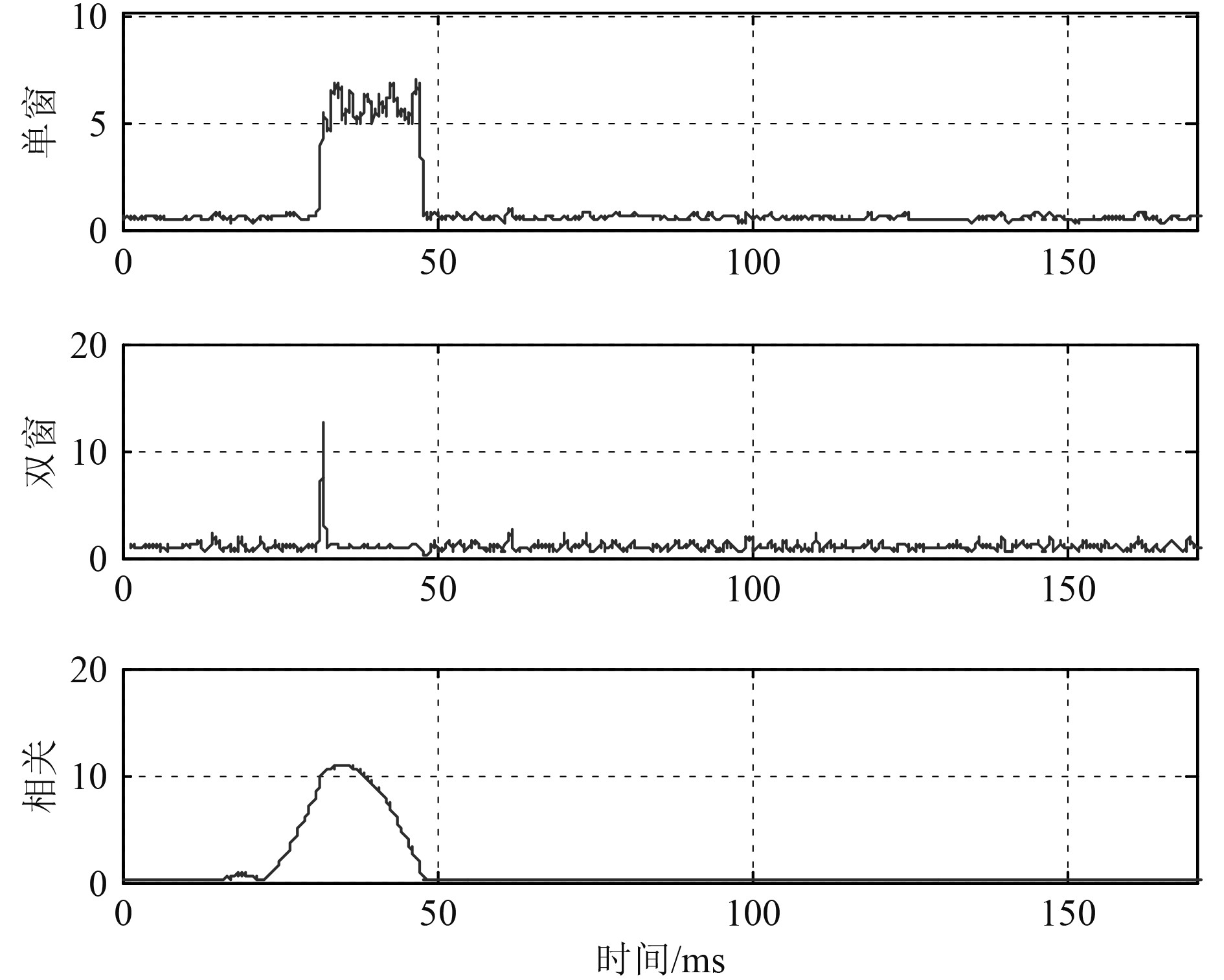图 2 脉冲信号检测输出（LFM，10 dB） Fig. 2 Detect for a LFM burst in 10 dB SNR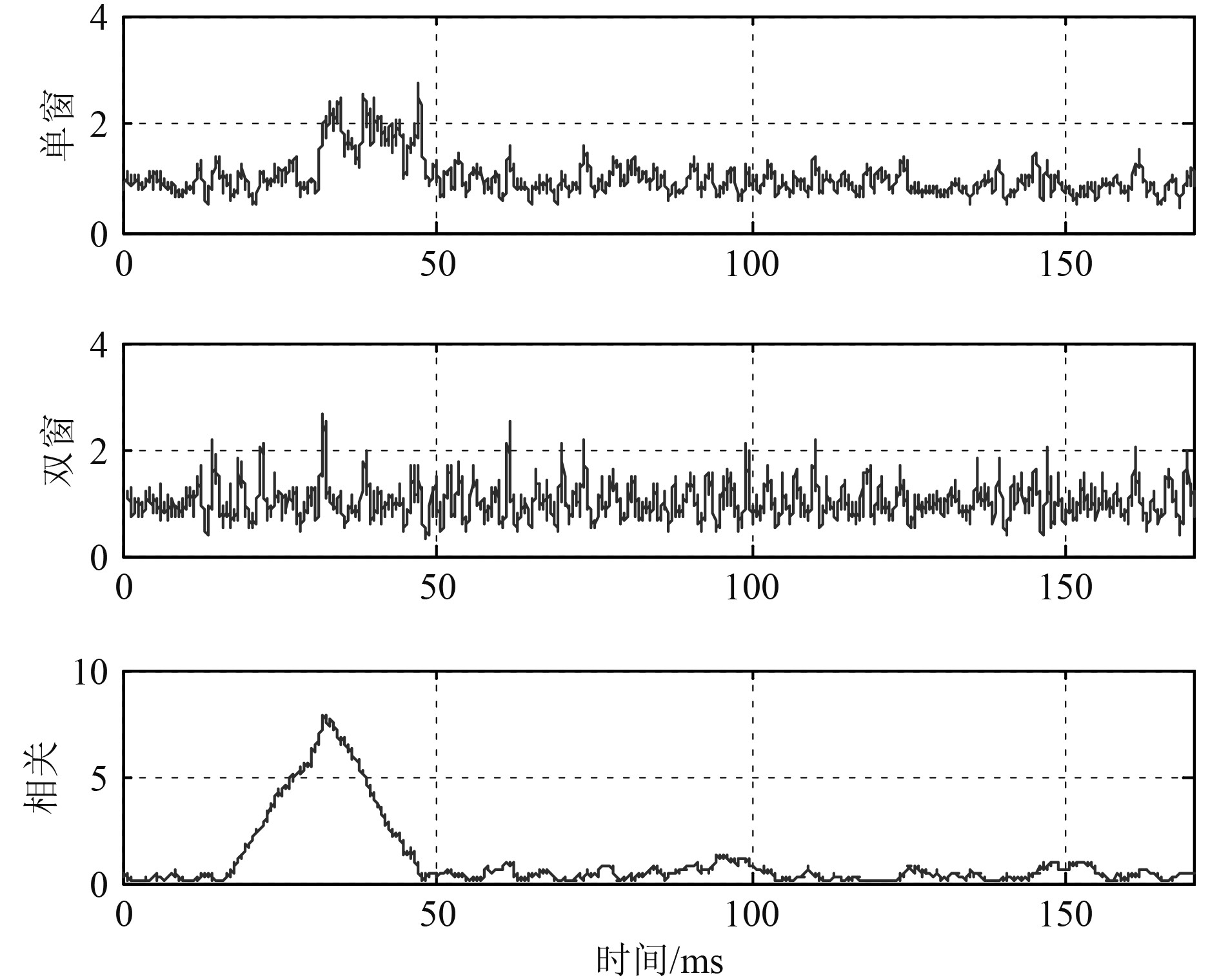图 3 脉冲信号检测输出（DSSS，0 dB） Fig. 3 Detect for a DSSS burst in 0 dB SNR

3）时频联合复本提取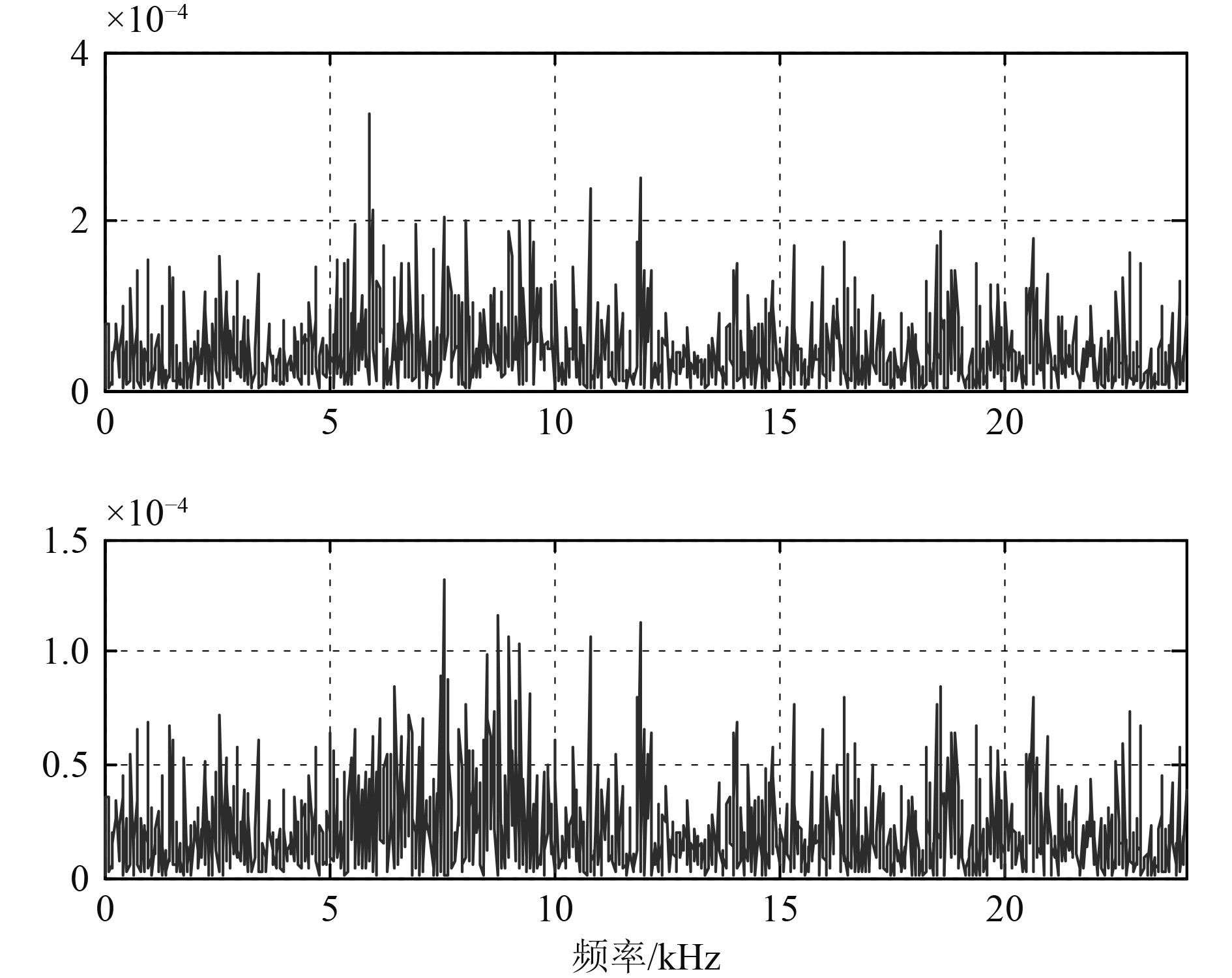图 4 LFM和DSSS脉冲信号功率谱密度 Fig. 4 Power spectral density of LFM and DSSS burst

 $STF{T_r}\left( {t,f} \right) = \int_{ - \infty }^{ + \infty } {r\left( \tau \right)w\left( {\tau - t} \right){e^{ - j2\text{π} f\tau }}{\rm{d}} \tau } 。$ (11)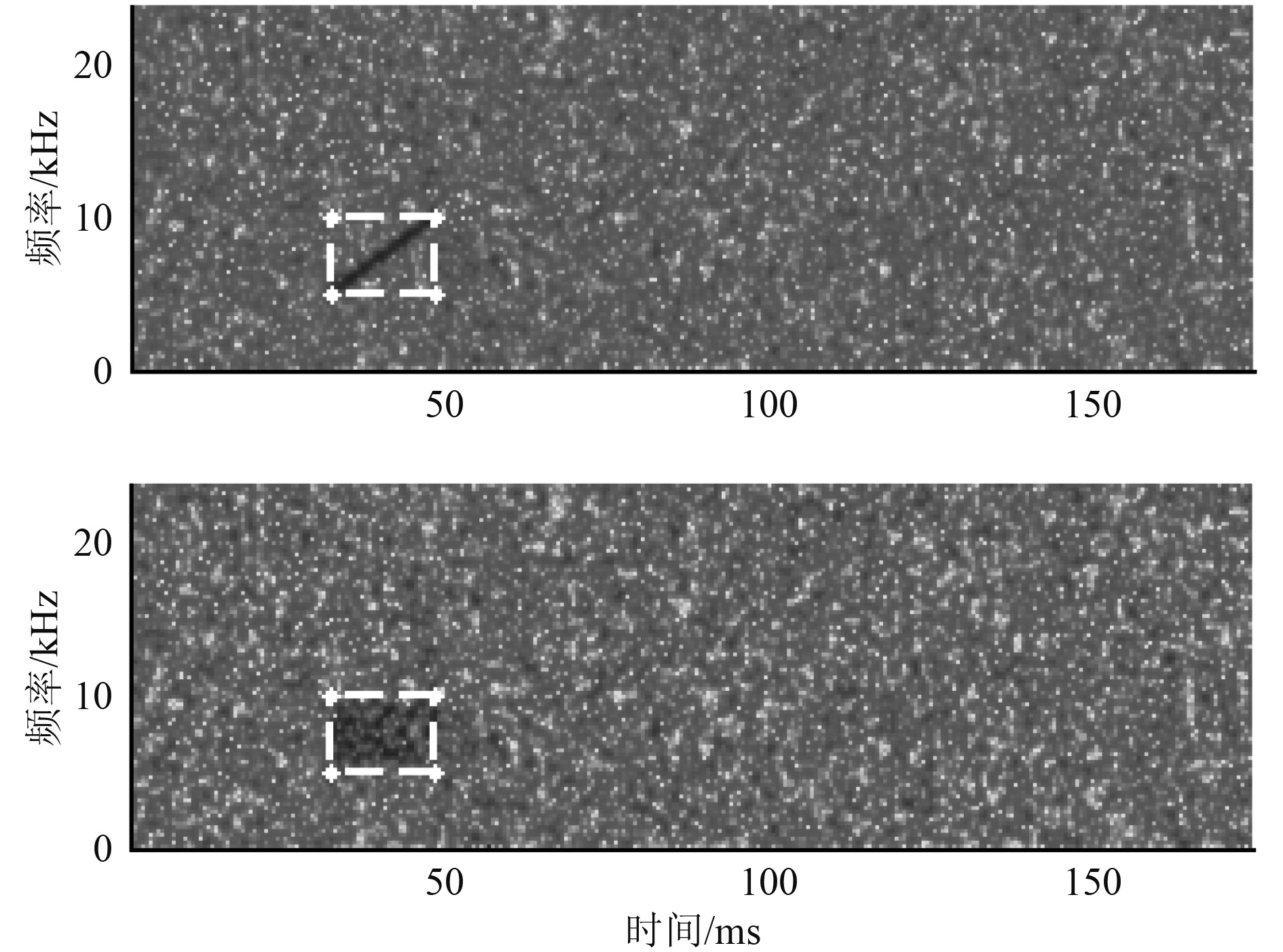图 5 LFM和DSSS脉冲信号STFT谱 Fig. 5 Spectrum of LFM and DSSS burst
1.4 匹配滤波

 $H\left( f \right) = c{S^*}\left( f \right){e^{ - j2\text{π} f\tau }}。$ (12)

 $G = 2TW 。$ (13)

2 仿真和试验验证 2.1 仿　真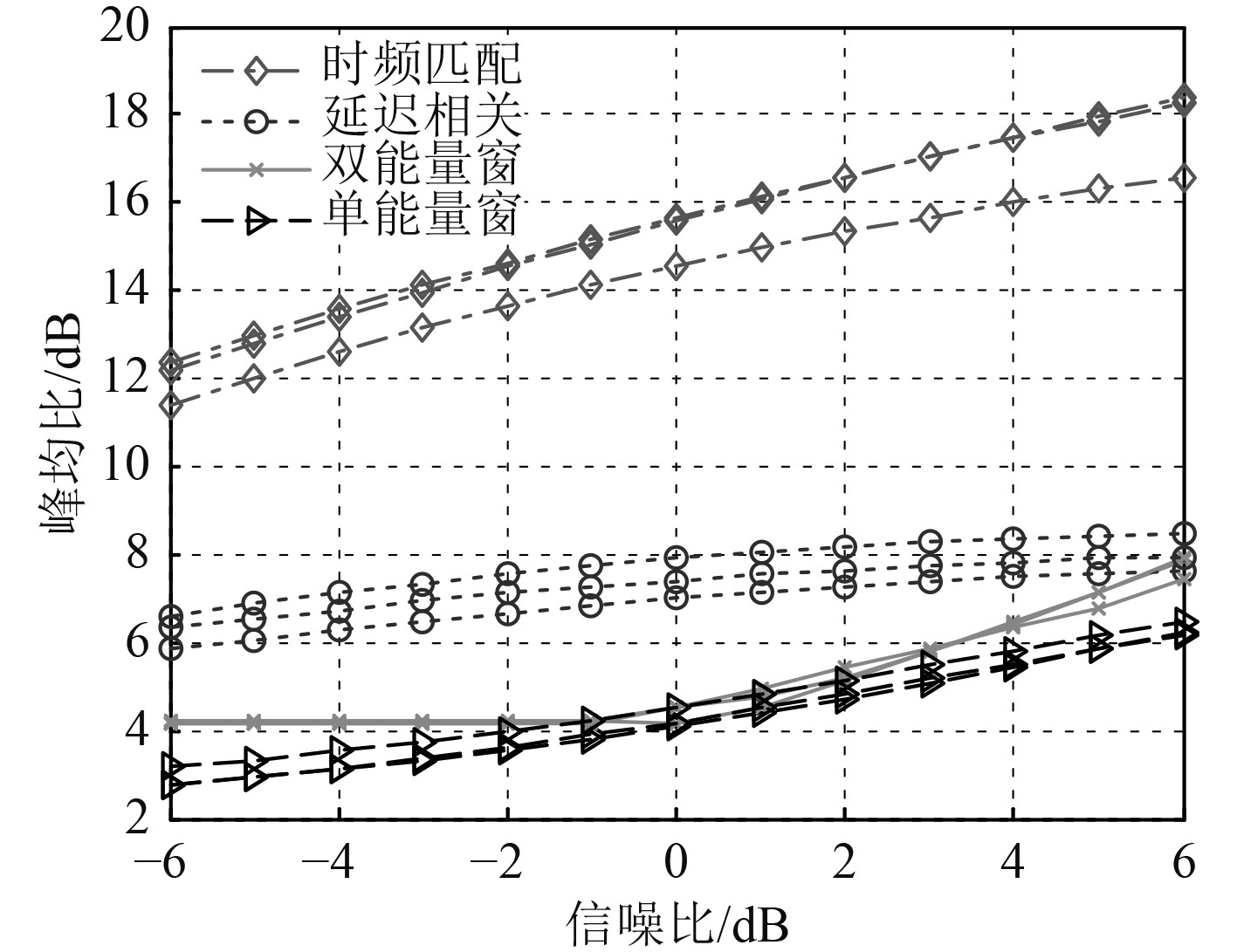图 6 几种检测算法的性能仿真曲线 Fig. 6 Performance Simulation of the detectors

2.2 湖试数据分析处理

1）单频脉冲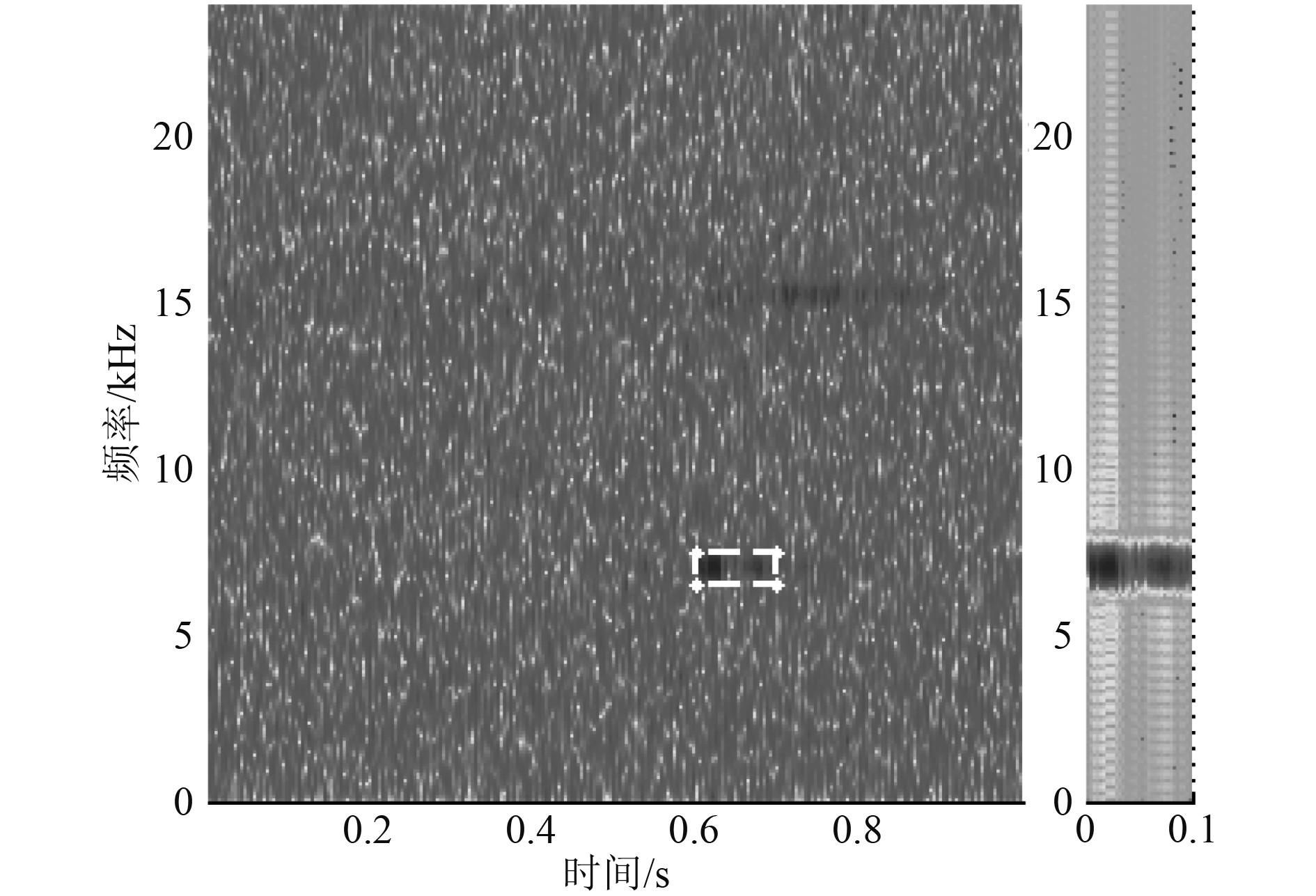图 7 时频联合复本提取 Fig. 7 Extract the burst in time-frequency domain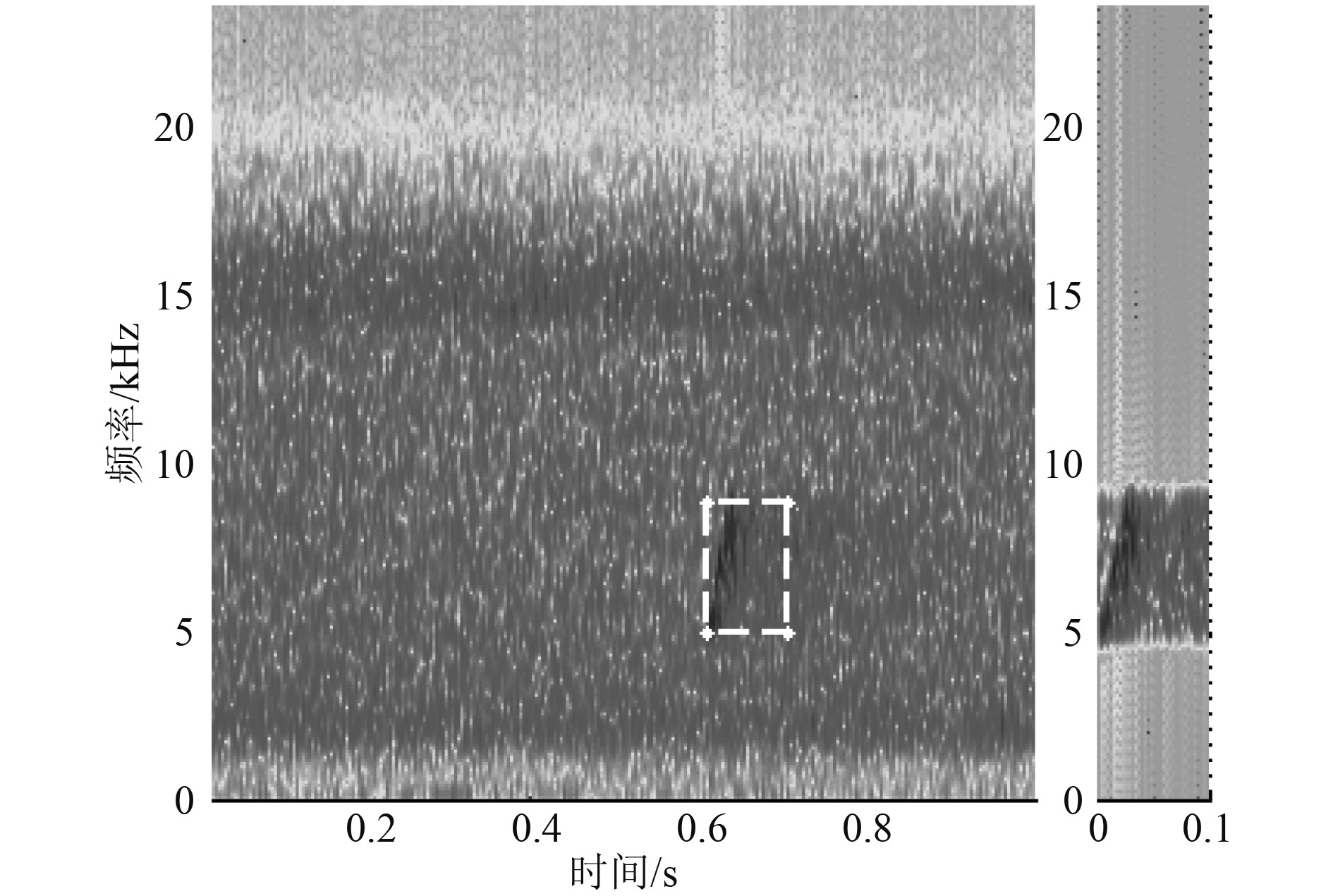图 8 各检测器输出峰均比曲线 Fig. 8 Output linear PARs of the detectors表 1 各检测器输出峰均比统计表 Tab.1 Linear PARs of detectors output

2）调频脉冲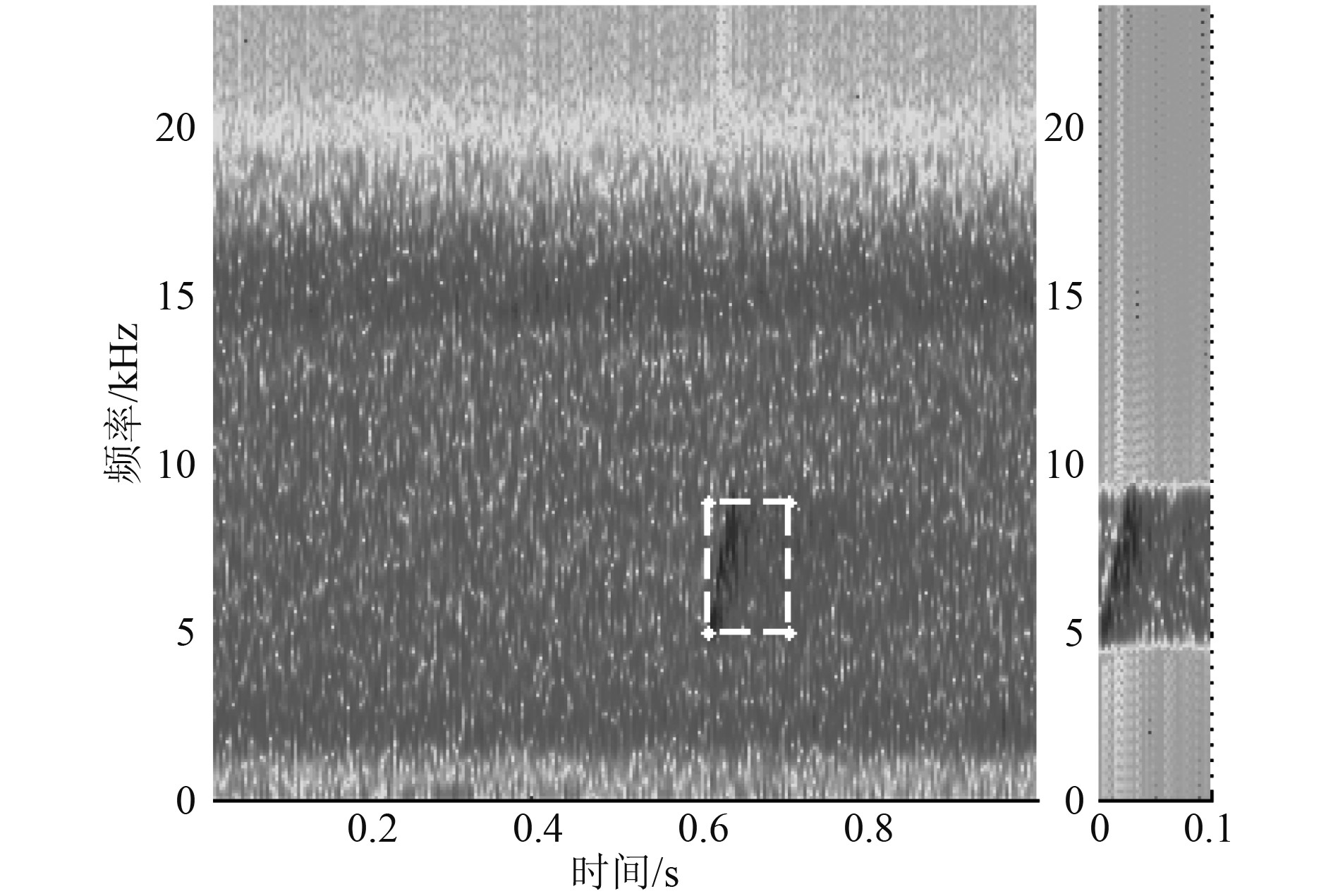图 9 时频联合复本提取 Fig. 9 Extract the burst in time-frequency domain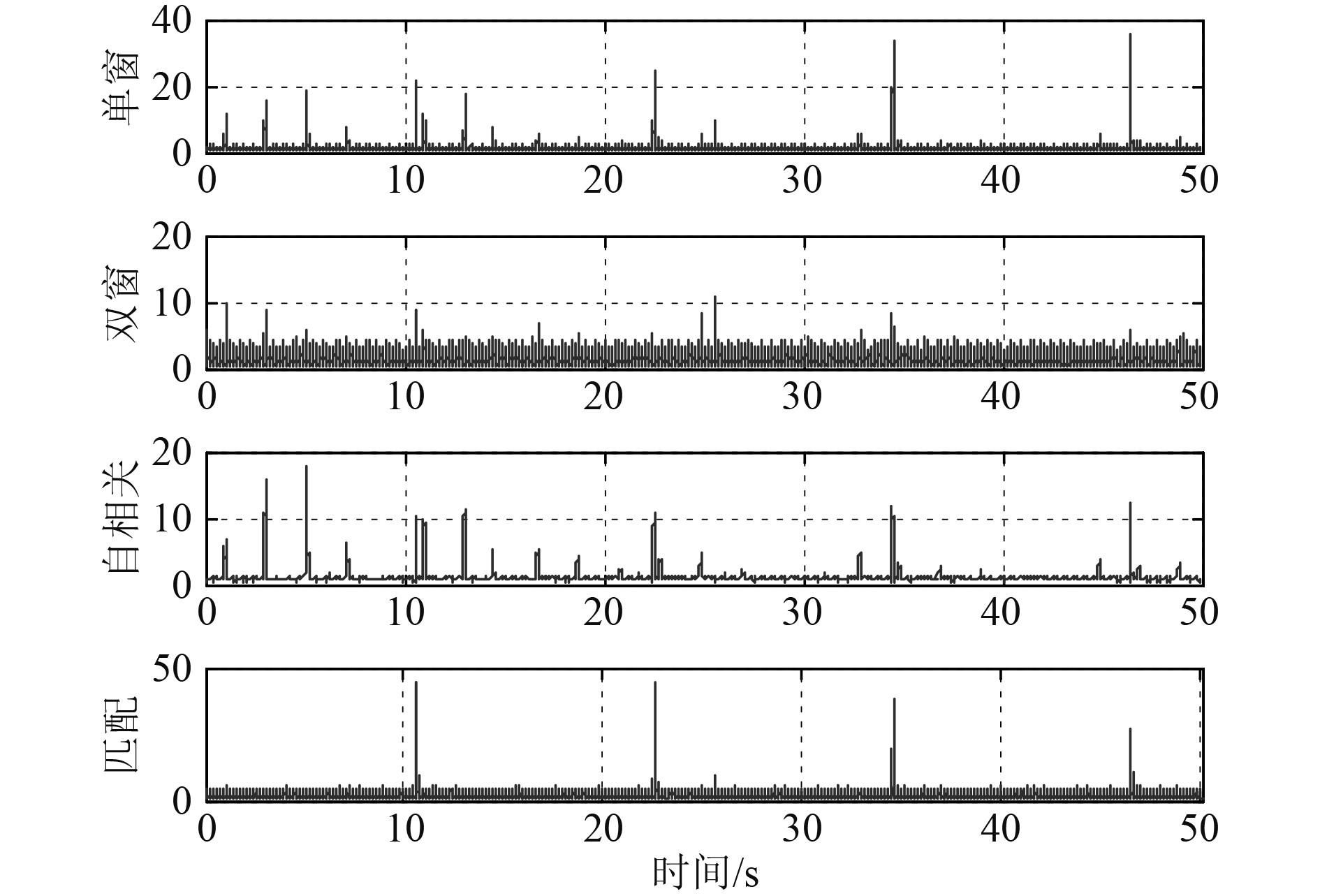图 10 各检测器输出峰均比曲线 Fig. 10 Output linear PARs of the detectors表 2 不同SNR下的正确检测情况统计表 Tab.2 Correctness of detecting for the following burst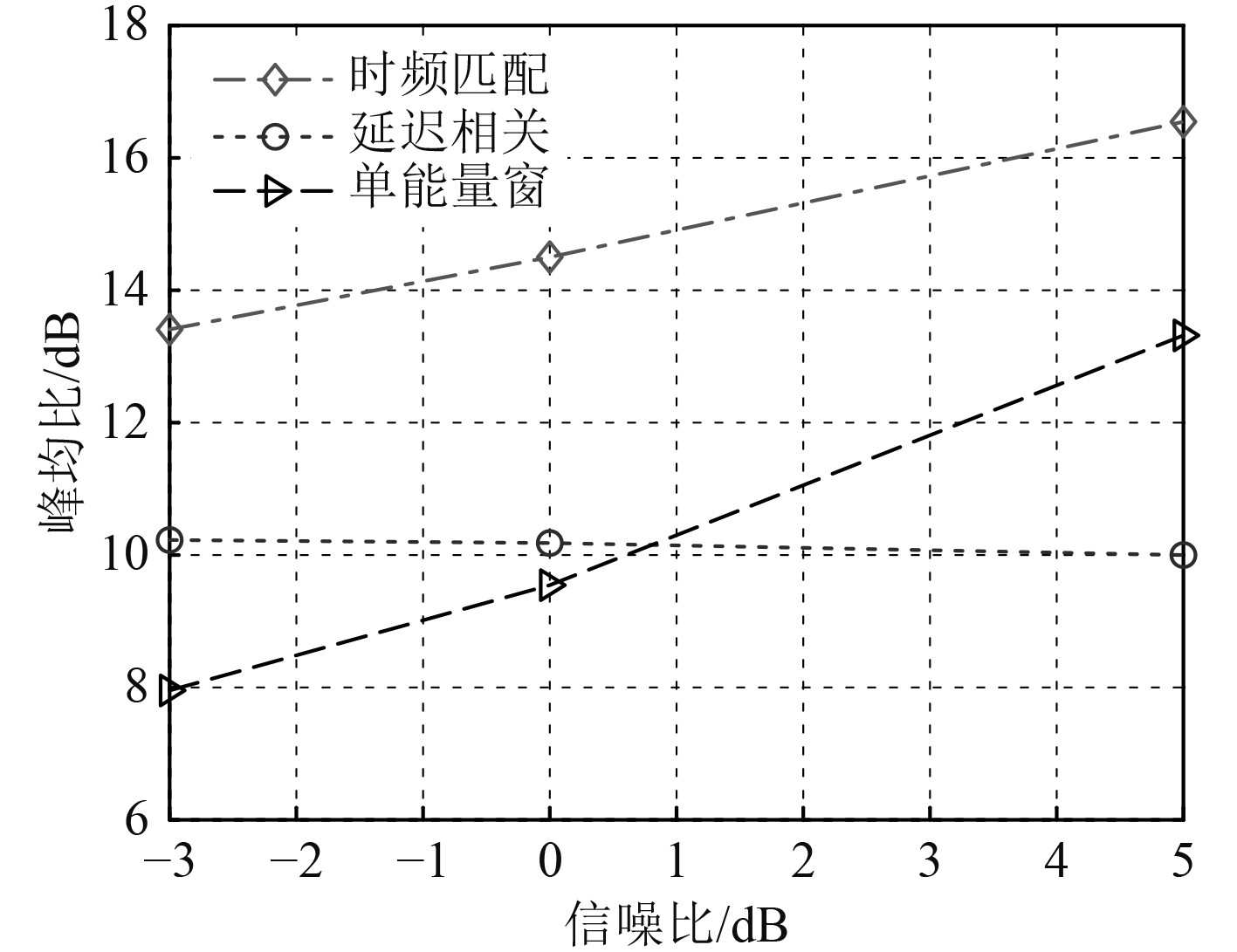图 11 对LFM脉冲数据盲检测的对数峰均比曲线 Fig. 11 Result of trial data processed with detectors in logarithmic PAR
3 结　语

  刘蓉, 霍甲. 信号盲检测应用情况简述[J]. 数字通信世界, 2014(6): 33-35.  王晓燕, 方世良, 朱志峰. 一种基于自相关估计的水声直扩信号检测方法[J]. 东南大学学报自然科学版, 2010, 40(2): 248-252. WANG Xiao-yan, FANG Shi-liang, ZHU Zhi-feng. Detection method of acoustic direct-sequence spread-spectrum signal based on autocorrelation estimation[J]. Journal of Southeast University (natural science edition), 2010, 40(2): 248-252.  张刚强, 董阳泽, 刘平香. 基于功率谱平均的水声直扩信号倒谱检测方法[J]. 哈尔滨工程大学学报, 2010, 31(7): 863-867. ZHANG Gang-qiang, DONG Yang-ze, LIU Ping-xiang. Detection of underwater acoustic DSSS signals using the cepstrum method based on a power spectral density average[J]. Jouranl of Harbin University, 2010, 31(7): 863-867.  谢绍斌, 周双, 王锋, 等. 基于滑动相关峰检测的混沌码同步法[J]. 电子与信息学报, 2010, 38(1): 141-145. XIE Shao-bin, ZHOU Shuang, WANG Feng, et al. Method of chaos code synchronization based on sliding correlation[J]. Journal of Electronics & Information Technology, 2010, 38(1): 141-145.  李启虎, 李敏, 杨秀庭. 水下目标辐射噪声中单频信号分量的检测: 理论分析[J]. 声学学报, 2008, 33(3): 193-196. LI Qi-hu, LI Min, YANG Xiu-ting. The detection of single frequency component of underwater radiated noise of target: theoretical analysis[J]. ACTA acoustic, 2008, 33(3): 193-196.  JUHA H, JOHN T. OFDM wireless LANs: a theoretical and practical guide [M]. Indiana of USA, Sams Publishing, 2002: 54–57.  刘佳敏, 赵知劲, 曹越飞, 等. 基于时频分析的多跳频信号盲检测[J]. 信号处理, 2021, 37(5): 763–771. LIU Jia-min, ZHAO Zhi-jin, CAO Yue-fei, et al. Blind detection of multi-frequency hopping signals based on time-frequency analysis[J]. Journal of Signal Processing, 2021, 37(5): 763–771.  周胜增, 杜选民. 被动声呐宽带检测与窄带检测的比较研究[J]. 声学技术, 2017, 36(5): 329–330. ZHOU Sheng-zeng, DU Xuan-min. Comparative research of wideband and narrowband for passive sonar[J]. Technical Acoustics, 2017, 36(5): 329–330.